Refer to our Texas Go Math Grade 1 Answer Key Pdf to score good marks in the exams. Test yourself by practicing the problems from Texas Go Math Grade 1 Module 2 Assessment Answer Key.

Vocabulary

Circle the number that is greater than 78. (p.64)
Underline the number that is less than 78. (p.70)

Question 1.
93 39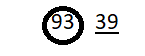Explanation:
93 > 78
39 < 78

Concepts and Skills

Write <, >, or =. TEKS 1.2.G, 1.2.E.

Question 2.
3850
Answer:38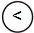50
Explanation:
38 is less than 50

Question 3.
1952
Answer: 1952
Explanation:
19 is less than 52

Question 4.
3535
Answer: 35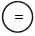35
Explanation:
35 is equals to 35

Question 5.
10099
Answer: 100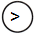99
Explanation:
100 is greater than 99

Question 6.
4443
Answer: 4443
Explanation:
44 is greater than 43

Question 7.
2662
Answer: 2662
Explanation:
26 is less than 62

Circle the greater number. Write the numbers. TEKS 1.2.G, 1.2.E

Question 8.
38 83
___ is greater than ____
___ > ____
Answer: 83  is greater than 38
83  > 38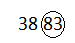Explanation:
circled the 83. Since 83  is greater than 38

Question 9.
Circle is greater than or is less than. Write a number to compare. TEKS 1.2.D, 1.2.E
102
is greater than is less than ___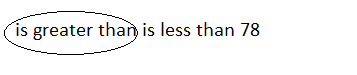Explanation:
78 is lesser than 102
112 is greater than 102

Texas Test Prep

Question 10.
Maria named a number less than 105. Which of these may be that number? TEKS 1.2.E
(A) 93
(B) 108
(C) 115
Explanation:
93 < 105
108 > 105
115> 105

Question 11.
Which symbol is needed? TEKS 1.2.G
98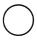98
(A) < (B) >
(C) =
Explanation:
9898
98  is equals to 98

Question 12.
Which number could belong at the dot? TEKS 1.2.F, 1.2.E(A) 98
(B) 88
(C) 78
Explanation:
88 is between 80 and 91 . 80 < 88< 91

Question 13.
Cami chooses a number greater than 64. Which shows a number she may choose? TEKS 1.2.E
(A) 64
(B) 58
(C) 72